## Minimum

The smallest value of a set, function, etc. The minimum value of a set of elements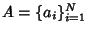is denoted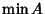or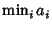, and is equal to the first element of a sorted (i.e., ordered) version of. For example, given the set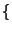3, 5, 4, 1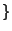, the sorted version is1, 3, 4, 5, so the minimum is 1. The Maximum and minimum are the simplest Order Statistics.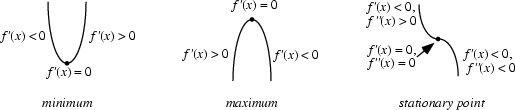A continuous Function may assume a minimum at a single point or may have minima at a number of points. A Global Minimum of a Function is the smallest value in the entire Range of the Function, while a Local Minimum is the smallest value in some local neighborhood.

For a function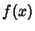which is Continuous at a point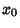, a Necessary but not Sufficient condition forto have a Relative Minimum at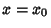is thatbe a Critical Point (i.e.,is either not Differentiable atoris a Stationary Point, in which case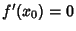).

The First Derivative Test can be applied to Continuous Functions to distinguish minima from Maxima. For twice differentiable functions of one variable,, or of two variables,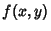, the Second Derivative Test can sometimes also identify the nature of an Extremum. For a function, the Extremum Test succeeds under more general conditions than the Second Derivative Test.

See also Critical Point, Extremum, First Derivative Test, Global Maximum, Inflection Point, Local Maximum, Maximum, Midrange, Order Statistic, Saddle Point (Function), Second Derivative Test, Stationary Point

References

Abramowitz, M. and Stegun, C. A. (Eds.). Handbook of Mathematical Functions with Formulas, Graphs, and Mathematical Tables, 9th printing. New York: Dover, p. 14, 1972.

Brent, R. P. Algorithms for Minimization Without Derivatives. Englewood Cliffs, NJ: Prentice-Hall, 1973.

Nash, J. C. Descent to a Minimum I-II: Variable Metric Algorithms.'' Chs. 15-16 in Compact Numerical Methods for Computers: Linear Algebra and Function Minimisation, 2nd ed. Bristol, England: Adam Hilger, pp. 186-206, 1990.

Press, W. H.; Flannery, B. P.; Teukolsky, S. A.; and Vetterling, W. T. Minimization or Maximization of Functions.'' Ch. 10 in Numerical Recipes in FORTRAN: The Art of Scientific Computing, 2nd ed. Cambridge, England: Cambridge University Press, pp. 387-448, 1992.

Tikhomirov, V. M. Stories About Maxima and Minima. Providence, RI: Amer. Math. Soc., 1991.